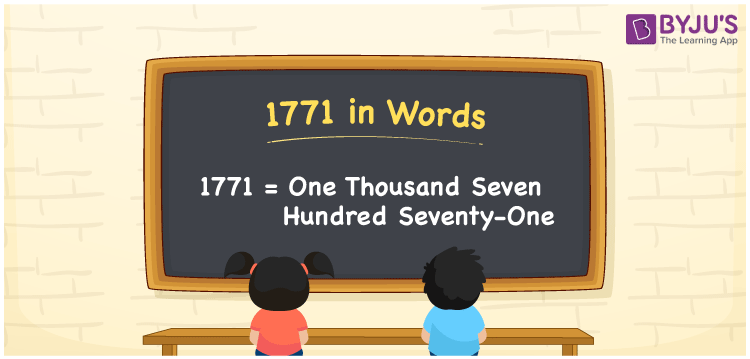# 1771 in words

1771 in words is written as One Thousand Seven Hundred and Seventy One. 1771 represents the count or value. The article on Counting Numbers can give you an idea about count or counting. The number 1771 is used in expressions that relate to money, distance, length, year and others. Let us consider an example for 1771. ”In the year One Thousand Seven Hundred and Seventy One, there was a plague outbreak”.

 1771 in words One Thousand Seven Hundred and Seventy One One Thousand Seven Hundred and Seventy One in Numbers 1771

## 1771 in English Words## How to Write 1771 in Words?

We can convert 1771 to words using a place value chart. The number 1771 has 4 digits, so let’s make a chart that shows the place value up to 4 digits.

 Thousands Hundreds Tens Ones 1 7 7 1

Thus, we can write the expanded form as:

1 × Thousand + 7 × Hundred + 7 × Ten + 1 × One

= 1 × 1000 + 7 × 100 + 7 × 10 + 1 × 1

= 1771

= One Thousand Seven Hundred and Seventy One.

1771 is the natural number that is succeeded by 1770 and preceded by 1772.

1771 in words – One Thousand Seven Hundred and Seventy One.

Is 1771 an odd number? – Yes.

Is 1771 an even number? – No.

Is 1771 a perfect square number? – No.

Is 1771 a perfect cube number? – No.

Is 1771 a prime number? – No.

Is 1771 a composite number? – Yes.

## Solved Example

1. Write the number 1771 in expanded form

Solution: 1 × 1000 + 7 × 100 + 7 × 10 + 1 × 1

We can write 1771 = 1000 +700 +70 + 1

= 1 × 1000 + 7 × 100 + 7 × 10 + 1 × 1

## Frequently Asked Questions on 1771 in words

Q1

### How to write 1771 in words?

1771 in words is written as One Thousand Seven Hundred and Seventy One.
Q2

### Is 1771 a perfect square number?

No. 1771 is not a perfect square number.
Q3

### Is 1771 a prime number?

No. 1771 is not a prime number.